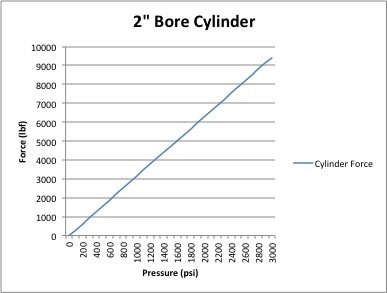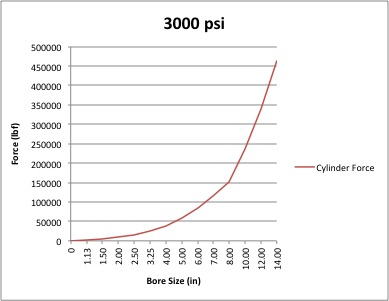Cylinders convert a fluid pressure into a mechanical force.  The input fluid pressure acts on the surface area of the cylinders piston displacing it to create a linear movement of the piston rod.

The output force of the cylinder is based on the pressure of the input fluid and surface area of the piston (which is driven by the cylinders bore diameter).

First let’s take a look at how pressure affects a cylinders force.  For this example we will compare a 2” bore pneumatic cylinder running on 90psi (common shop air pressure) and a 2” bore hydraulic cylinder running on 3000psi (common hydraulic pump pressure).

Since both cylinders have the same bore diameter their piston surface area will be the same.  A quick calculation for determining the surface area is as follows:

For extend area :  .7854 x (bore diameter)^2

For retract area:   .7854 x ((bore diameter)^2 - (rod diameter)^2)

So for our 2” bore cylinders the extend area would be .7854 x (2”)^2 = 3.14 sq. in.

Now that we have the piston surface area we can multiply it by our cylinders input pressures to get our output forces.

For the pneumatic cylinder 90 psi x 3.14 sq. in. = 282 lbf.

For the hydraulic cylinder 3000 psi x 3.14 sq. in. = 9420 lbf.As shown on the graph with a fixed bore size input pressure forms a linear relationship with the output force.

For the next example let’s look at how changing the bore size affects the output force.

Using the same 2” bore hydraulic cylinder running on 3000psi from the example above let’s compare it to a 4” bore cylinder with the same pressure.

From the previous example the 2” bore cylinder has an extend area of 3.14 sq. in. x 3000 psi = 9420 lbf.

The 4” bore cylinder has an extend area of .7854 x (4)^2 = 12.56 sq. in.

This gives us an output force of 12.56 sq. in. x 3000 psi = 37680 lbf.

An easy way to understand this relationship when sizing a hydraulic system, when you double the bore size of a cylinder you get four times the output force on the same input pressure.

Here is a graph of the most common NFPA hydraulic cylinder bore sizes and their output force based on a 3000 psi pressure input.Without "force" hydraulic systems just wouldn't work. A little late we know but, may the force (or May the fourth) be with you.

Image credit: Wikimedia CommonsTopics: Application